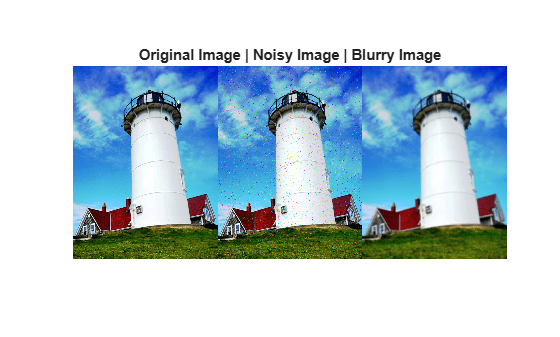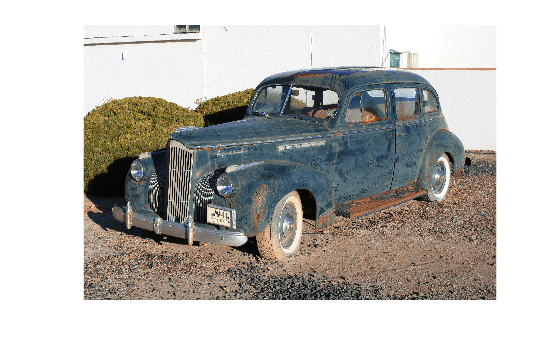# niqe

Naturalness Image Quality Evaluator (NIQE) no-reference image quality score

## Syntax

``score = niqe(A)``
``score = niqe(A,model)``

## Description

example

````score = niqe(A)` calculates the no-reference image quality score for image `A` using the Naturalness Image Quality Evaluator (NIQE). `niqe` compares `A` to a default model computed from images of natural scenes. A smaller score indicates better perceptual quality.```

example

````score = niqe(A,model)` calculates the image quality score using a custom model.```

## Examples

collapse all

Compute the NIQE score for a natural image and its distorted versions using the default model.

Read an image into the workspace. Create copies of the image with noise and blurring distortions.

```I = imread('lighthouse.png'); Inoise = imnoise(I,'salt & pepper',0.02); Iblur = imgaussfilt(I,2);```

Display the images.

```figure montage({I,Inoise,Iblur},'Size',[1 3]) title('Original Image | Noisy Image | Blurry Image')```Calculate the NIQE score for each image using the default model. Display the score.

```niqeI = niqe(I); fprintf('NIQE score for original image is %0.4f.\n',niqeI)```
```NIQE score for original image is 2.5455. ```
```niqeInoise = niqe(Inoise); fprintf('NIQE score for noisy image is %0.4f.\n',niqeInoise)```
```NIQE score for noisy image is 10.8770. ```
```niqeIblur = niqe(Iblur); fprintf('NIQE score for blurry image is %0.4f.\n',niqeIblur)```
```NIQE score for blurry image is 5.2661. ```

The original undistorted image has the best perceptual quality and therefore the lowest NIQE score.

Load a set of natural images into an image datastore. These images are shipped in Image Processing Toolbox™ in a directory named 'imdata'.

```setDir = fullfile(toolboxdir('images'),'imdata'); imds = imageDatastore(setDir,'FileExtensions',{'.jpg'});```

Train a custom NIQE model using the image datastore.

`model = fitniqe(imds);`
```Extracting features from 33 images. .. Completed 7 of 33 images. Time: Calculating... .. Completed 11 of 33 images. Time: 00:23 of 01:35 ...... Done. ```

Read an image of a natural scene. Display the image.

```I = imread('car1.jpg'); imshow(I)```Calculate the NIQE score for the image using the custom model. Display the score.

```niqeI = niqe(I,model); fprintf('NIQE score for the image is %0.4f.\n',niqeI)```
```NIQE score for the image is 2.6700. ```

## Input Arguments

collapse all

Input image, specified as a 2-D grayscale or RGB image.

Data Types: `single` | `double` | `int16` | `uint8` | `uint16`

Custom model of image features, specified as a `niqeModel` object. `model` is derived from natural scene statistics.

## Output Arguments

collapse all

No-reference image quality score, returned as a nonnegative scalar. Lower values of `score` reflect better perceptual quality of image `A` with respect to the input `model`.

Data Types: `double`

## Algorithms

NIQE measures the distance between the NSS-based features calculated from image `A` to the features obtained from an image database used to train the model. The features are modeled as multidimensional Gaussian distributions.

 Mittal, A., R. Soundararajan, and A. C. Bovik. "Making a Completely Blind Image Quality Analyzer." IEEE Signal Processing Letters. Vol. 22, Number 3, March 2013, pp. 209–212.